Win up to 100% scholarship on Aakash BYJU'S JEE/NEET courses with ABNAT Win up to 100% scholarship on Aakash BYJU'S JEE/NEET courses with ABNAT

# JEE Main 2022 July 29 – Shift 1 Chemistry Question Paper with Solutions

The JEE Main 2022 July 29 – Shift 1 Chemistry Question Paper with Solutions is given on this page. JEE Main 2022 answer keys help students to make a self-assessment regarding their performance in the JEE Main exam. Those who are preparing for the upcoming JEE Main exams can use the JEE Main 2022 question paper with solutions to get an idea of the pattern and the difficulty level. Practising the JEE Main 2022 question papers is an effective tool for time management. Check out the JEE Main 2022 July 29 – Shift 1 Chemistry Question Paper with Solutions given below.

## JEE Main 2022 29th July Shift 1 Chemistry Question Paper and Solutions

SECTION – A

Multiple Choice Questions: This section contains 20 multiple choice questions. Each question has 4 choices (1), (2), (3) and (4), out of which ONLY ONE is correct.

1. Which of the following pair of molecules contain odd electron molecule and an expanded octet molecule?

(A) BCl3 and SF6

(B) NO and H2SO4

(C) SF6 and H2SO4

(D) BCl3 and NO

Sol. NO is an odd electron species as N has 5 valence electrons and O has 6 valence electrons. Thus overall 1 electron on N remains unpaired.

S in H2SO4 has an expanded octet thus H2SO4 is expanded octet molecule.

2.

$$\begin{array}{l} \underset{20\text{ g}}{N_{\text{2(g)}}}+\underset{5\text{ g}}{3H_{2\text{(g)}}}\rightleftharpoons 2NH_{3\text{(g)}}\end{array}$$

Consider the above reaction, the limiting reagent of the reaction and number of moles of NH3 formed, respectively are :

(A) H2, 1.42 moles

(B) H2, 0.71 moles

(C) N2, 1.42 moles

(D) N2, 0.71 moles

Sol.

$$\begin{array}{l} \underset{20\text{ g}}{N_{\text{2(g)}}}+\underset{5\text{ g}}{3H_{2\text{(g)}}}\rightleftharpoons 2NH_{3\text{(g)}}\end{array}$$

Ideally 28 g N2 reacts with 6 g H2 limiting reagent is N2

∴ Amount of NH3 formed on reacting 20 g N2 is,

$$\begin{array}{l} =\frac{34\times20}{28}=24.28\text{ g} \end{array}$$

= 1.42 moles

3. 100 mL of 5% (w/v) solution of NaCl in water was prepared in 250 mL beaker. Albumin from the egg was poured into NaCl solution and stirred well. This resulted in a/an :

(A) Lyophilic sol

(B) Lyophobic sol

(C) Emulsion

(D) Precipitate

Sol. Albumin from the egg was poured into 100 mL of 5% (w/v) NaCl solution in water. This would result in the formation of lyophilic sol. Albumin molecules get dispersed in water the colloidal particles of albumin are stabilised by hydrogen bond with water molecules.

4. The first ionization enthalpy of Na, Mg and Si, respectively, are : 496, 737 and 786 kJ mol–1. The first ionization enthalpy (kJ mol–1) of Al is:

(A) 487

(B) 768

(C) 577

(D) 856

Sol. The first ionisation enthalpy of Al would be more than that of (sodium) Na but less than that of (silicon) Si and (magnesium) Mg.

Thus, first ionisation enthalpy of Al would be 577 kJ/mole.

5. In metallurgy the term “gangue” is used for :

(A) Contamination of undesired earthy materials.

(B) Contamination of metals, other than desired metal.

(C) Minerals which are naturally occurring in pure form

(D) Magnetic impurities in an ore.

Sol. The term “gangue” is used for earthy or undesired materials in ore.

6. The reaction of zinc with excess of aqueous alkali, evolves hydrogen gas and gives :

(A) Zn(OH)2

(B) ZnO

(C) [Zn(OH)4]2–

(D) [ZnO2]2–

Sol. Reaction of zinc with excess of aqueous alkali evolving hydrogen gas is as

$$\begin{array}{l} Zn+2NaOH\xrightarrow[~~~~]{}\underset{\text{Sodium Zincate}}{Na_2ZnO_2}+\underset{\text{Hydrogen gas}}{H_2}\end{array}$$

Thus along with H2 it gives Na2ZnO2 or ZnO22-.

7. Lithium nitrate and sodium nitrate, when heated separately, respectively, give :

(A) LiNO2 and NaNO2

(B) Li2O and Na2O

(C) Li2O and NaNO2

(D) LiNO2 and Na2O

Sol. Lithium nitrate when heated gives lithium oxide (Li2O) whereas sodium nitrate on heating gives sodium nitrite

4LiNO3→ 2Li2O + 4NO2 + O2

2NaNO3→ 2NaNO2 + O2

8. Number of lone pairs of electrons in the central atom of SCl2, O3, CIF3 and SF6, respectively, are:

(A) 0, 1, 2 and 2

(B) 2, 1, 2 and 0

(C) 1, 2, 2 and 0

(D) 2, 1, 0 and 2

Sol. The number of lone pair of electrons in the central atom of SCl2, O3, CIF3 and SF6 are 2, 1, 2 and O respectively

Their structures are as,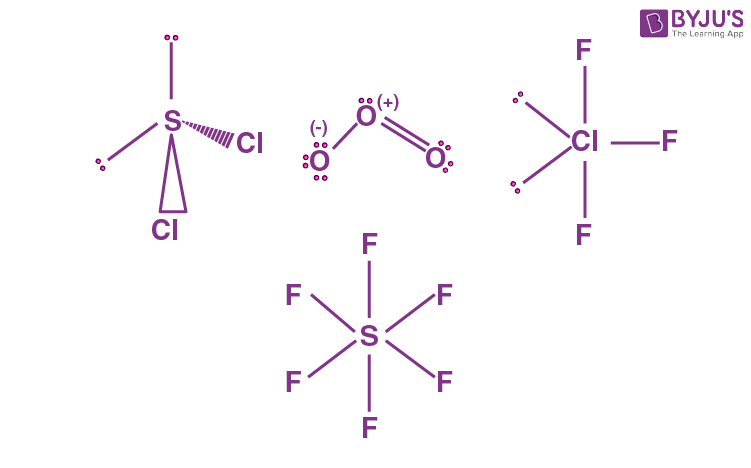9. In following pairs, the one in which both transition metal ions are colourless is :

(A) Sc3+, Zn2+

(B) Ti4+, Cu2+

(C) V2+, Ti3+

(D) Zn2+, Mn2+

Sol. Sc+3 and Zn+2are colourless as they contain no unpaired electron. Whereas the transition metal ions Cu+2, Ti+3, V+2 and Mn+2are coloured as they contain unpaired electrons.

The unpaired electron from lower energy d orbital gets excited to a higher energy d orbital on absorbing light of frequency which lies in visible region. The colour complementary to light absorbed is observed.

10. In neutral or faintly alkaline medium, KMnO4 being a powerful oxidant can oxidize, thiosulphate almost quantitatively, to sulphate. In this reaction overall change in oxidation state of manganese will be

(A) 5

(B) 1

(C) 0

(D) 3

Sol. In neutral or Faintly alkaline medium,

thiosulphate is oxidised almost quantitatively to sulphate ion according to reaction given below,

$$\begin{array}{l} 8MnO_4^-+3S_2O_3^{2-}+H_2O\rightarrow 8MnO_2+6SO_4^{2-}+2OH^- \end{array}$$

Here the Mn changes from Mn+7 to Mn+4

Thus, overall change in its oxidation number would be of 3.

11. Which among the following pairs has only herbicides?

(A) Aldrin and Dieldrin

(B) Sodium chlorate and Aldrin

(C) Sodiumarsinate and Dieldrin

(D) Sodium chlorate and sodiumarsinite

Sol. Aldrin and Dieldrin are examples of pesticides, whereas Sodium chlorate (NaClO3) and Sodium arsinite(Na3ASO3) are examples of herbicides.

12. Which among the following is the strongest Bronsted base?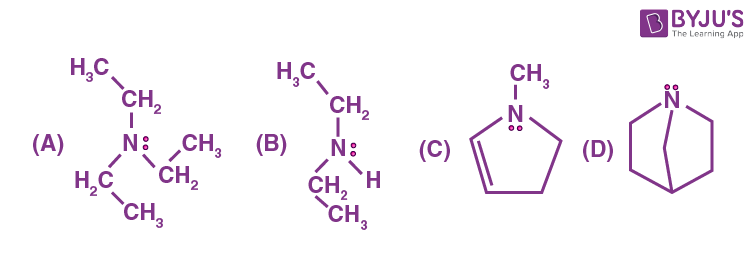Sol.is the strongest base among the given compounds due to the maximum +I effect and the lone pair of N is not in dynamic state so it can be donated easily.

13. Which among the following pairs of the structures will give different products onozonolysis? (Consider the double bonds in the structures are rigid and not delocalized)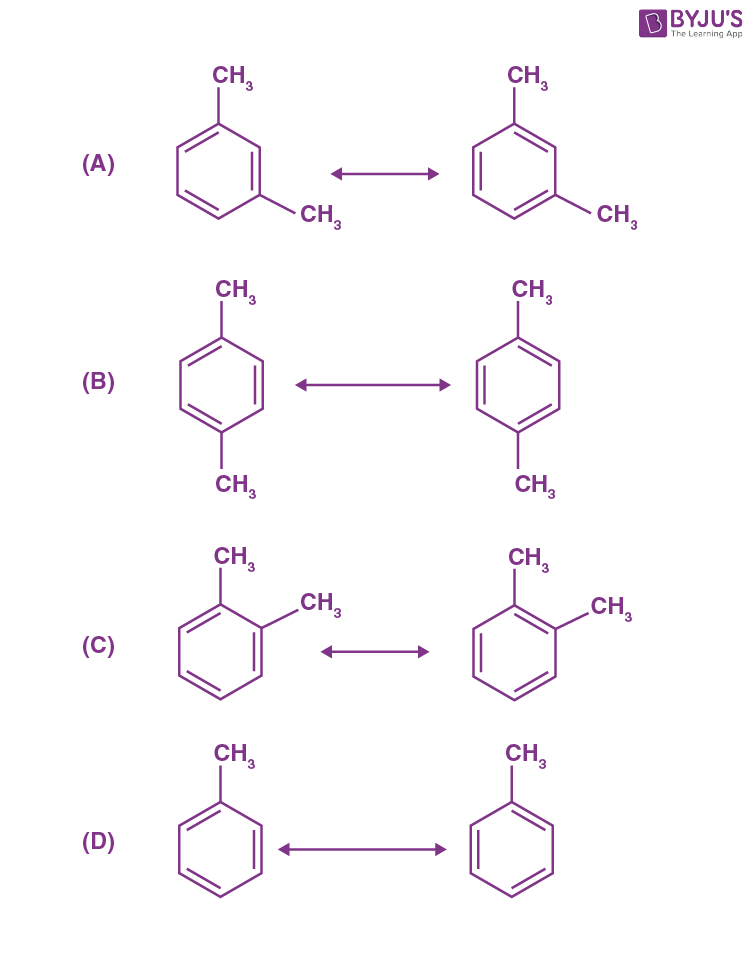Sol.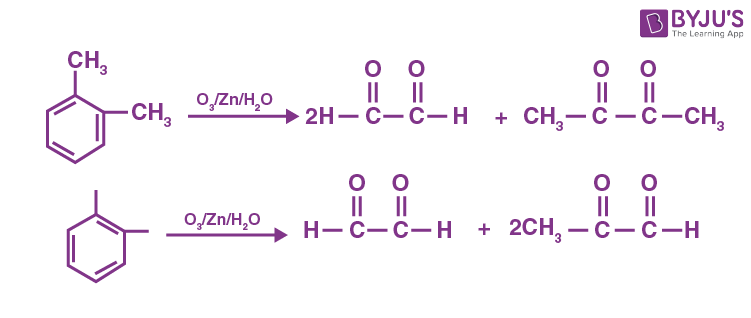∴ In option (C), different products are produced.

14.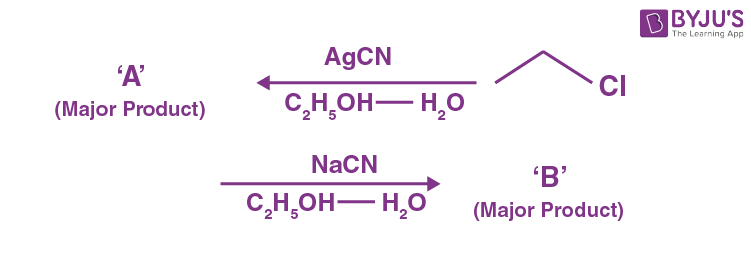Considering the above reactions, the compound ‘A’ and compound ‘B’ respectively are :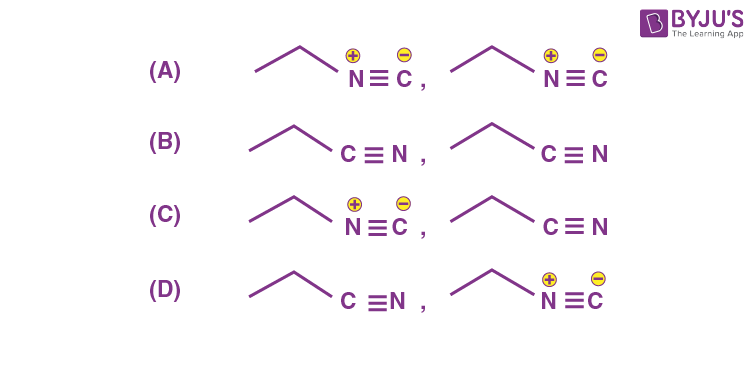Sol.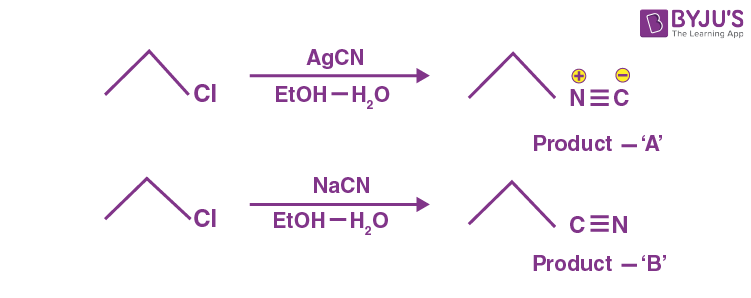$$\begin{array}{l}\text{KCN is ionic so}\ \overset{\ominus }{C}N\ \text{attacks through ‘C’ – atom.}\end{array}$$

AgCN is covalent so CN attacks through ‘N’ – atom.

15.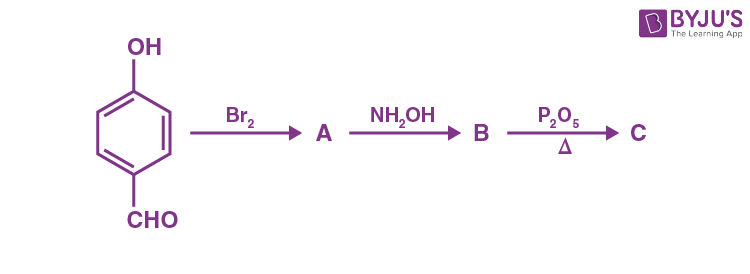Consider the above reaction sequence, the product ‘C’ is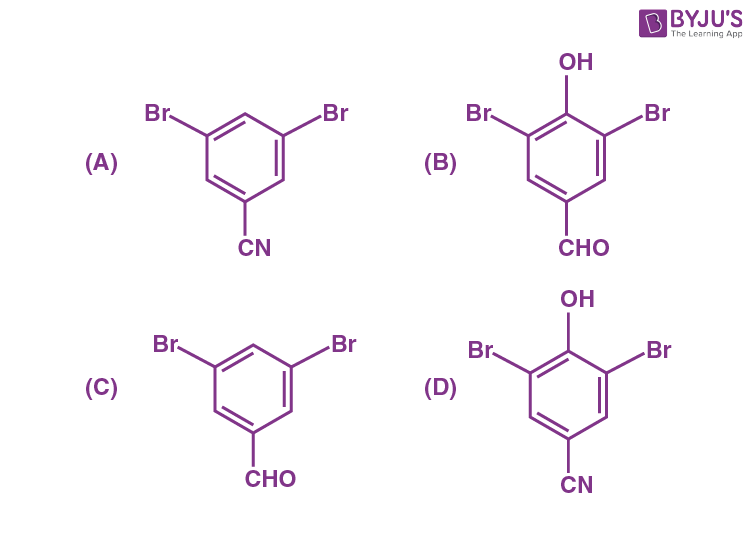Sol.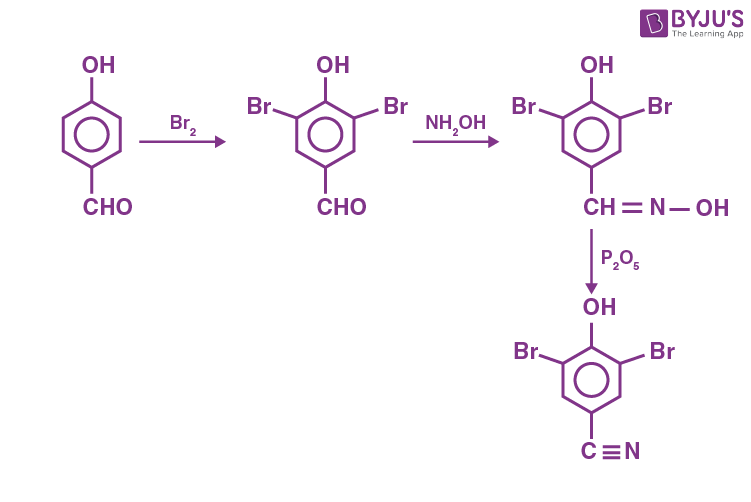16.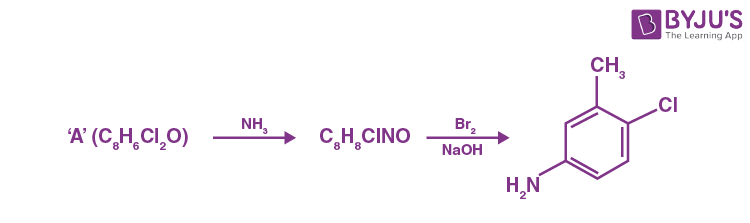Consider the above reaction, the compound ‘A’ is :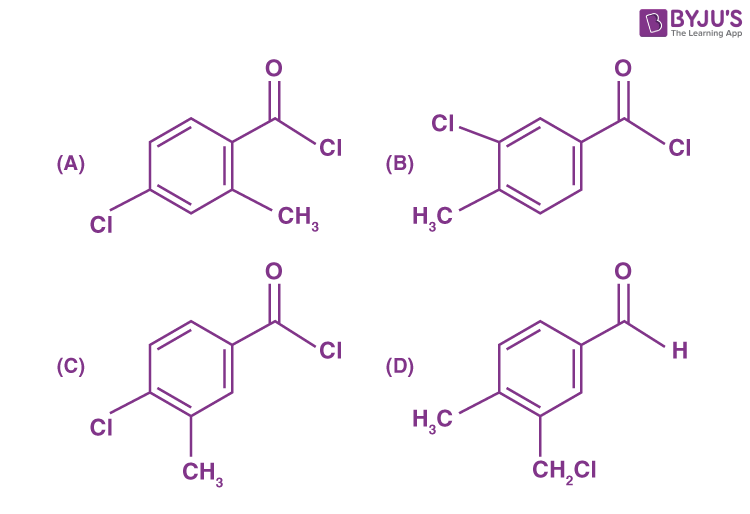Sol.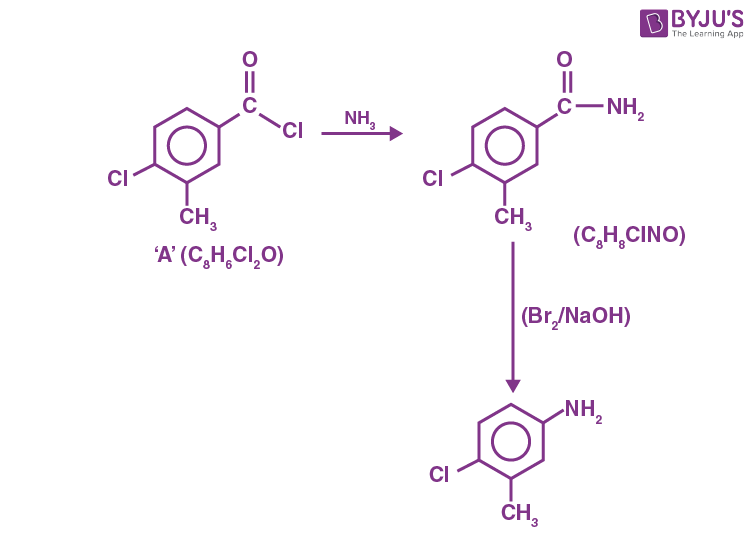17.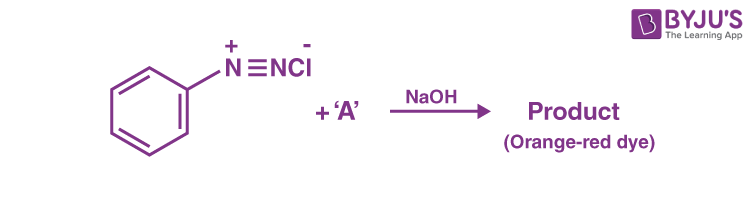Which among the following represents reagent ‘A’?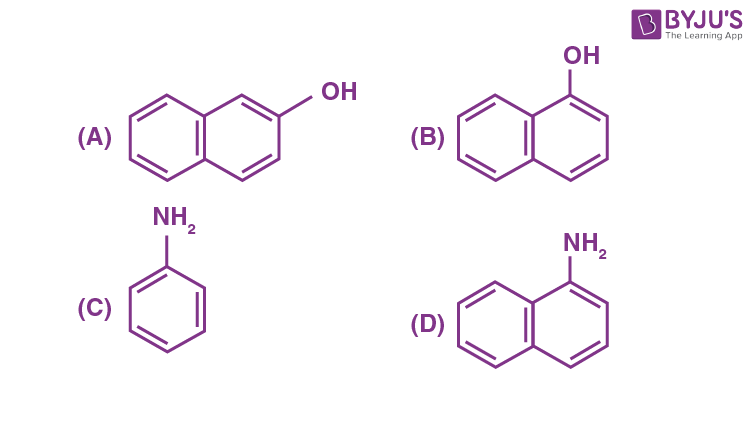Sol.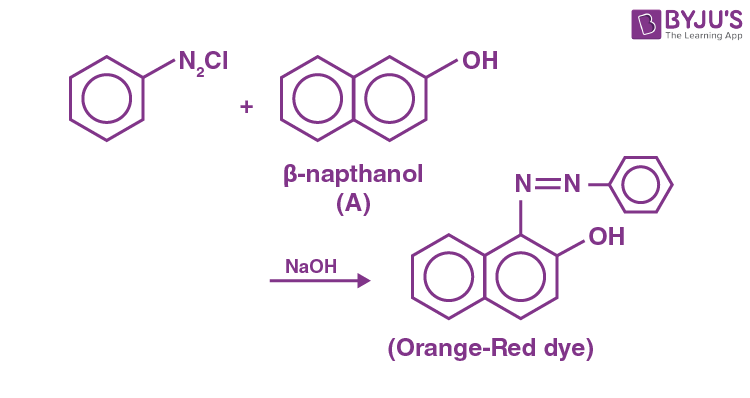18. Consider the following reaction sequence :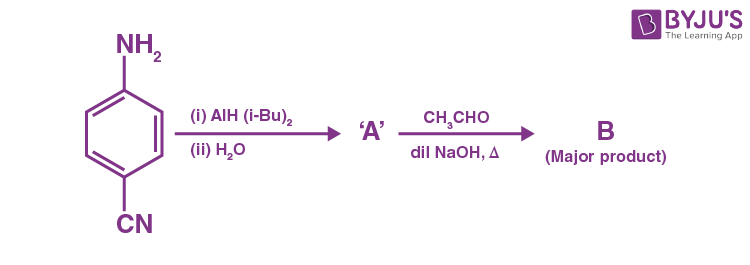The product ‘B’ is :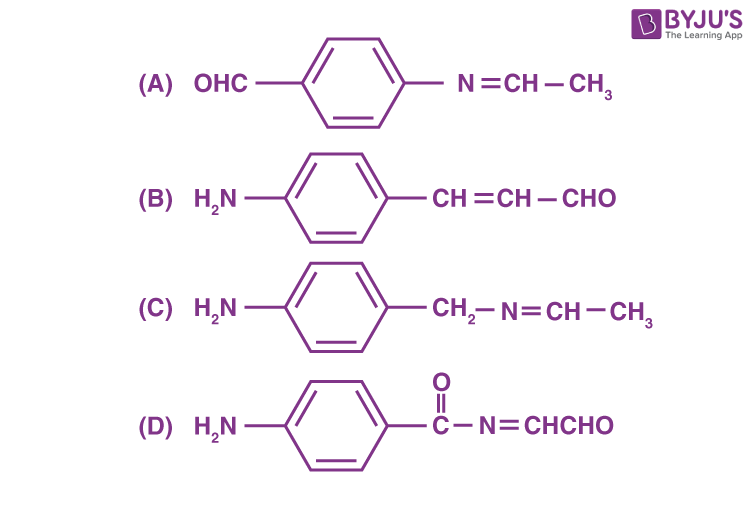Sol.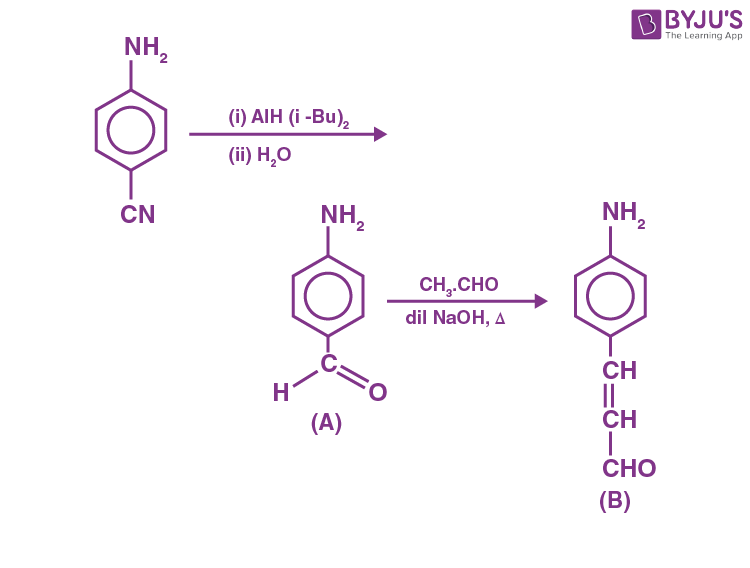2nd reaction is the cross aldol reaction.

19. Which of the following compounds is an example of hypnotic drug?

(A) Seldane

(B) Amytal

(C) Aspartame

(D) Prontosil

Sol. Seldane→ Antihistamine

Amytal → Barbiturate (Hypnotic)

Aspartame → Artificial sweetener

Prontosil → Antibiotics

20. A compound ‘X’ is acidic and it is soluble in NaOH solution, but insoluble in NaHCO3 solution. Compound ‘X’ also gives violet colour with neutral FeCl3 solution. The compound ‘X’ is :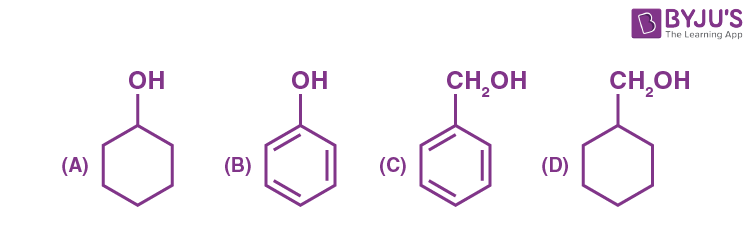Sol.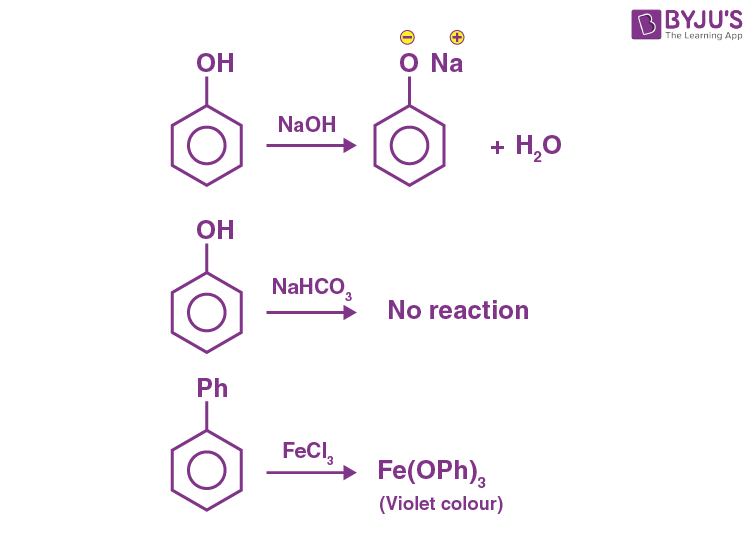SECTION – B

Numerical Value Type Questions: This section contains 10 questions. In Section B, attempt any five questions out of 10. The answer to each question is a NUMERICAL VALUE. For each question, enter the correct numerical value (in decimal notation, truncated/rounded-off to the second decimal place; e.g. 06.25, 07.00, –00.33, –00.30, 30.27, –27.30) using the mouse and the on-screen virtual numeric keypad in the place designated to enter the answer.

1. Resistance of a conductivity cell (cell constant 129 m–1) filled with 74.5 ppm solution of KCI is 100 Ω (labelled as solution 1). When the same cell is filled with KCl solution of 149 ppm, the resistance is 50 Ω (labelled as solution 2). The ratio of molar conductivity of solution 1 and solution 2 is

$$\begin{array}{l} \frac{\Lambda_1}{\Lambda_2}=x\times 10^{-3}.\end{array}$$
The value of x is _________. (Nearest integer)

(Given : molar mass of KCl is 74.5 g mol–1).

Sol. Solution 1,

$$\begin{array}{l} \Lambda_{m_1}=\frac{1000K}{M}\end{array}$$
$$\begin{array}{l} M=\frac{74.5}{74.5}\times\frac{1000}{10^6}=10^{-3}\text{ M}\end{array}$$
[density of solution = 1 g/mol]
$$\begin{array}{l} \Lambda_1=\frac{1000\times 129\times10^{-4}}{10^{-3}}=129\times10^2\text{ Scm}^2\text{ mol}^{-1}\end{array}$$
$$\begin{array}{l} \left[K=\frac{x}{R}=\frac{129\times10^{-2}}{100}\right]\end{array}$$

Solution 2,

$$\begin{array}{l} K=\frac{129\times10^{-2}}{50} \end{array}$$
$$\begin{array}{l} \Lambda_2=\frac{1000\times129\times10^{-2}}{50 M} \end{array}$$
$$\begin{array}{l} M=\frac{149}{74.5}\times \frac{1000}{10^6}=2\times10^{-3}\text{ M}\end{array}$$
$$\begin{array}{l} \Lambda_2=\frac{1000\times129\times10^{-2}}{50\times2\times10^{-3}}=129\times10^2\text{ Scm}^2\text{ mol}^{-1} \end{array}$$
$$\begin{array}{l} \frac{\Lambda_1}{\Lambda_2}=1=1000\times10^{-3} \end{array}$$

⇒ x = 1000

2. Ionic radii of cation A+ and anion B are 102 and 181 pm, respectively. These ions are allowed to crystallize into an ionic solid. This crystal has cubic close packing for B. A+ is present in all octahedral voids. The edge length of the unit cell of the crystal AB is _____pm. (Nearest integer)

Sol.

In cubic close packing, octahedral voids form at edge centers and body center of the cube

a = 2(rA+ +rB)

a = 2 (102 + 181)

a = 566 pm

3. The minimum uncertainty in the speed of an electron in an one dimensional region of length 2a0 (Where a0 = Bohr radius 52.9 pm) is _____ km s–1.

(Given : Mass of electron = 9.1 × 10–31 kg, Planck’s constant h = 6.63 × 10–34Js)

Sol.

$$\begin{array}{l} \Delta x\cdot\Delta v\geq \frac{h}{4\pi m} \end{array}$$

Δx = 2 × 52.9 × 10–12 m

$$\begin{array}{l} \Delta v\geq\frac{6.63\times10^{-34}}{4\times3.14\times9.1\times10^{-31}\times2\times52.9\times10^{-12}} \end{array}$$
$$\begin{array}{l} \Delta v\geq5.48\times 10^{-4}\times 10^9\text{ m/s} \end{array}$$
$$\begin{array}{l} \Delta v\geq 548\text{ km/s}\end{array}$$
(Rounded off to the nearest integer)

4. When 600 mL of 0.2 M HNO3 is mixed with 400 mL of 0.1 M NaOH solution in a flask, the rise in temperature of the flask is ________ × 10–2 °C.

(Enthalpy of neutralisation = 57 kJ mol–1 and Specific heat of water = 4.2 JK–1 g–1)

Sol. H+ + OH→ H2O

m moles 120 40 –

80 – 40

Heat liberated from reaction

= 40 × 10–3× 57 × 103 J …(1)

Heat gained by solution = mCΔT

m = mass of solution = V × d = 1000 × 1

= 1000 g

Heat gained by solution = 1000 × 4.2 × ΔT …(2)

From (1) and (2)

Heat liberated = Heat gained

40 × 10–3 × 57 × 103 = 1000 × 4.2 × ΔT

ΔT = 54 × 10–2 °C

(Rounded off to the nearest integer)

5. If O2 gas is bubbled through water at 303 K, the number of millimoles of O2 gas that dissolve in 1 litre of water is_______. (Nearest integer)

(Given : Henry’s Law constant for O2 at 303 K is 46.82 k bar and partial pressure of O2 = 0.920 bar)

(Assume solubility of O2 in water is too small, nearly negligible)

Sol. From Henry’s law,

$$\begin{array}{l} X\left(\text{oxygen}\right)=\frac{p\left(\text{oxygen}\right)}{K_H}=\frac{0.920}{46.82\times 10^3}=1.96\times 10^{-5}\end{array}$$

As 1 litre of water contains 55.5 mol of it, therefore,

→ n represents moles of O2 in solution.

$$\begin{array}{l} X\left(\text{oxygen}\right)=\frac{n}{n+55.5}\simeq \frac{n}{55.5}\left(n<<55.5\right) \end{array}$$
$$\begin{array}{l} \frac{n}{55.5}=1.96\times 10^{-5}\end{array}$$

n = 108.8 × 10–5 = 1.08 ×10–3 moles

m moles of oxygen = 1.08 × 10–3 × 103= 1 m mole

6. If the solubility product of PbS is 8 × 10–28, then the solubility of PbS in pure water at 298 K is x × 10–16 mol L–1. The value of x is ________. (Nearest integer)

$$\begin{array}{l}\left[Given : \sqrt{2} = 1.41\right] \end{array}$$

Sol.

$$\begin{array}{l} PbS\left(s\right)\rightleftharpoons P\underset{S}{b}^{2+}\left(aq\right)+S_S^{2-}\left(aq\right)\end{array}$$

Ksp = S2

8 × 10–28 = S2

$$\begin{array}{l} S=2\sqrt{2}\times 10^{-14}\text{ mol/L}\end{array}$$

2.82×10–14mol/L = 282×10–16 mol/L

Hence, x = 282

7. The reaction between X and Y is first order with respect to X and zero order with respect to Y.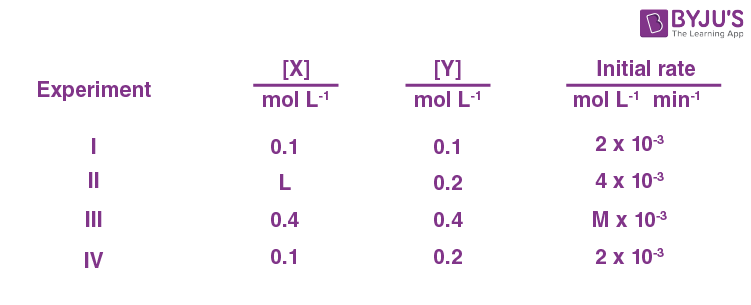Examine the data of table and calculate ratio of numerical values of M and L.(Nearest integer)

Sol. Rate [X]1[Y]0

Rate = k[X]

From Exp I and II,

$$\begin{array}{l} \frac{4\times10^{-3}}{2\times10^{-3}}=\left(\frac{L}{0.1}\right)^1\left(\frac{0.2}{0.1}\right)^0 \end{array}$$

2 = (10 L)1.

Hence L = 0.2 mol/L

From Exp III and IV,

$$\begin{array}{l} \frac{M\times10^{-3}}{2\times10^{-3}}=\left(\frac{0.4}{0.1}\right)\left(\frac{0.4}{0.2}\right)^0\end{array}$$
$$\begin{array}{l} \frac{M}{2}=4 \end{array}$$

M = 8

$$\begin{array}{l} \frac{M}{L}=\frac{8}{0.2}=40\end{array}$$

8. In a linear tetrapeptide (constituted with different amino acids) – (number of peptide bonds) is _____.

Sol. In a linear tetrapeptide, four amino acids are linked and three peptide bonds are present.

Hence, 4 – 3 = 1

9. In bromination of Propyne, with Bromine 1,1,2,2-tetrabromopropane is obtained in 27% yield. The amount of 1,1,2,2-tetrabromopropane obtained from 1 g of Bromine in this reaction is
_______ × 10–1 g. (Nearest integer)

(Molar Mass : Bromine = 80 g/mol)

Sol.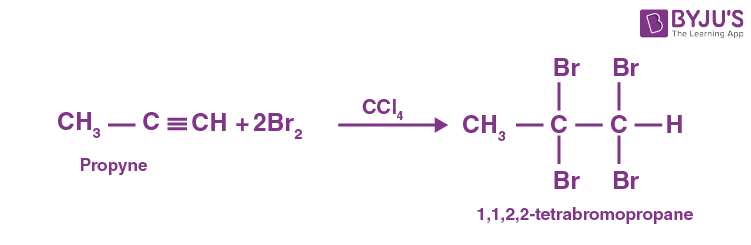2 moles Br2≡1 mole 1,1,2,2-tetrabromopropane

1/160 mole Br2

$$\begin{array}{l} \equiv\frac{1}{2}\times\frac{1}{160} \end{array}$$
mole 1,1,2,2-tetrabromopropane

But yield of reaction is only 27%

Moles of 1,1,2,2-tetrabromopropane

$$\begin{array}{l} =\frac{1}{2}\times \frac{1}{160}\times\frac{27}{100}\end{array}$$

Molar mass of 1,1,2,2-tetrabromopropane = 360 g

Mass of 1,1,2,2-tetrabromopropane

$$\begin{array}{l} =\frac{1}{2}\times\frac{1}{160}\times\frac{27}{100}\times360\text{ g}\end{array}$$

= 3 × 10–1 g

10. [Fe(CN)6]3– should be an inner orbital complex. Ignoring the pairing energy, the value of crystal field stabilization energy for this complex is (–) _____ Δ0. (Nearest integer)

Sol.

In [Fe(CN)6]3–, Fe is present in (+3) oxidation state Fe(III) ⇒ inner orbital complex ⇒ d 5(with pairing)

$$\begin{array}{l}\text{Configuration}\ \Rightarrow t_{2g}^5\end{array}$$
$$\begin{array}{l} \text{CFSE}=5\times\frac{-2}{5}\Delta_0=-2\Delta_0 \end{array}$$

### Download PDF of JEE Main 2022 July 29 Shift 1 Chemistry Paper & Solutions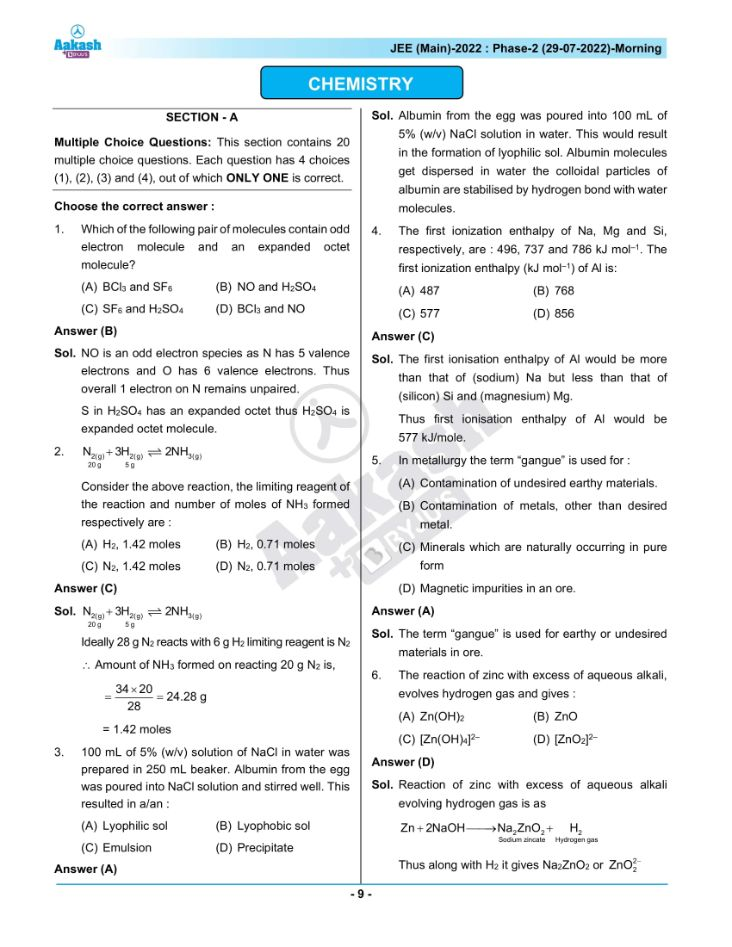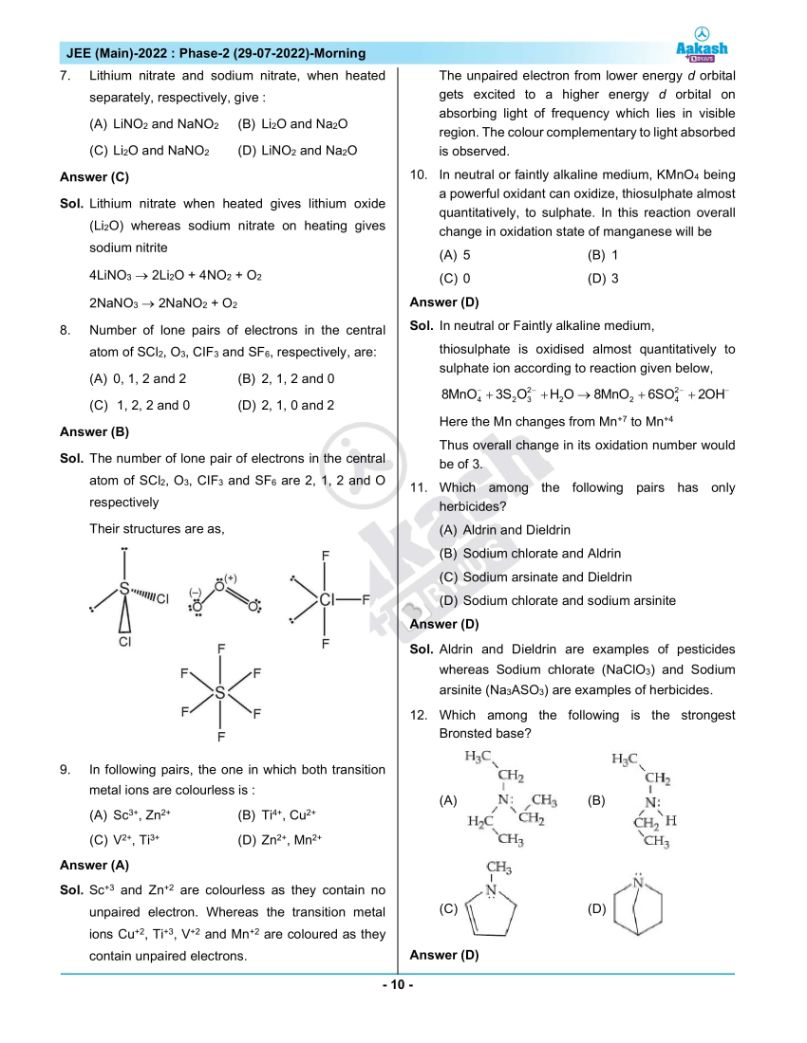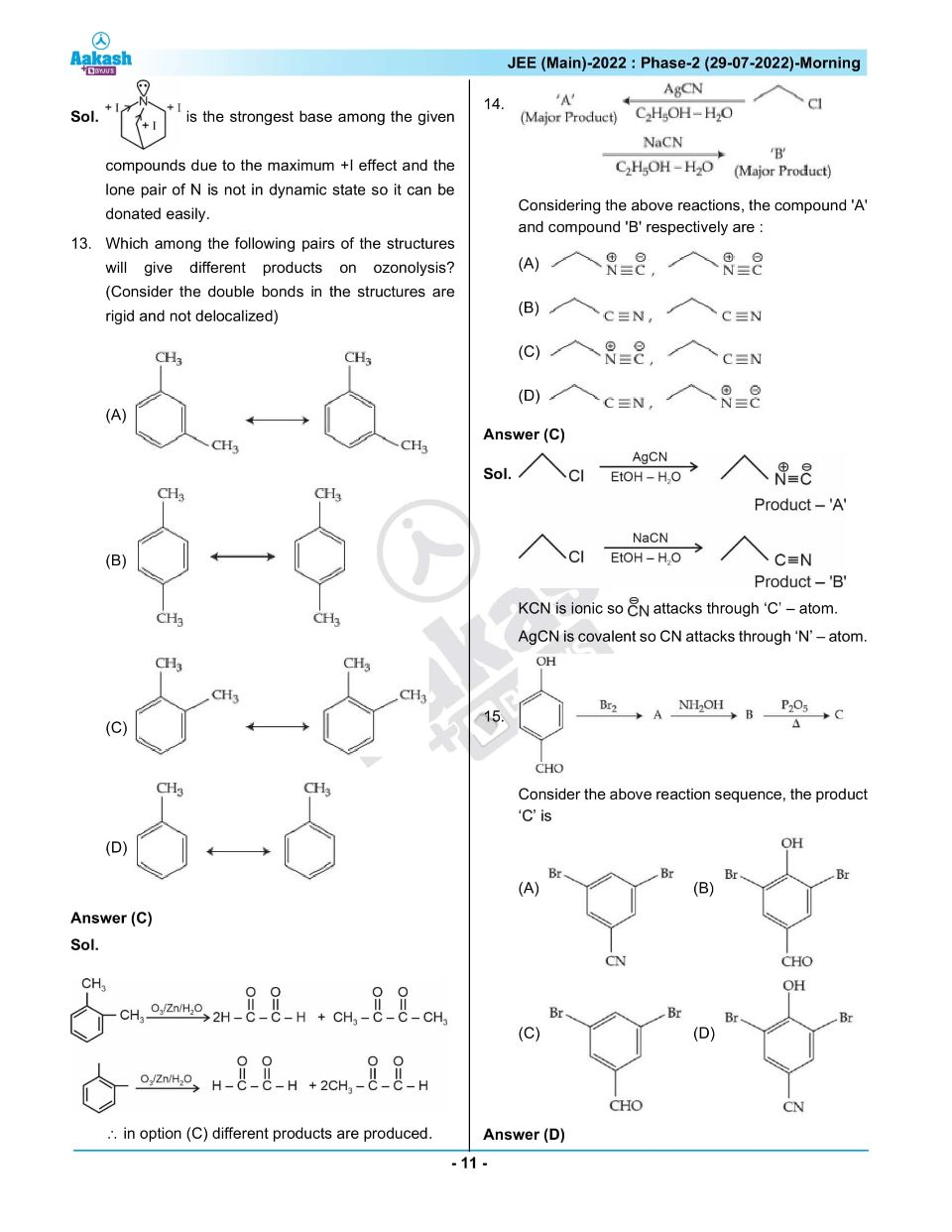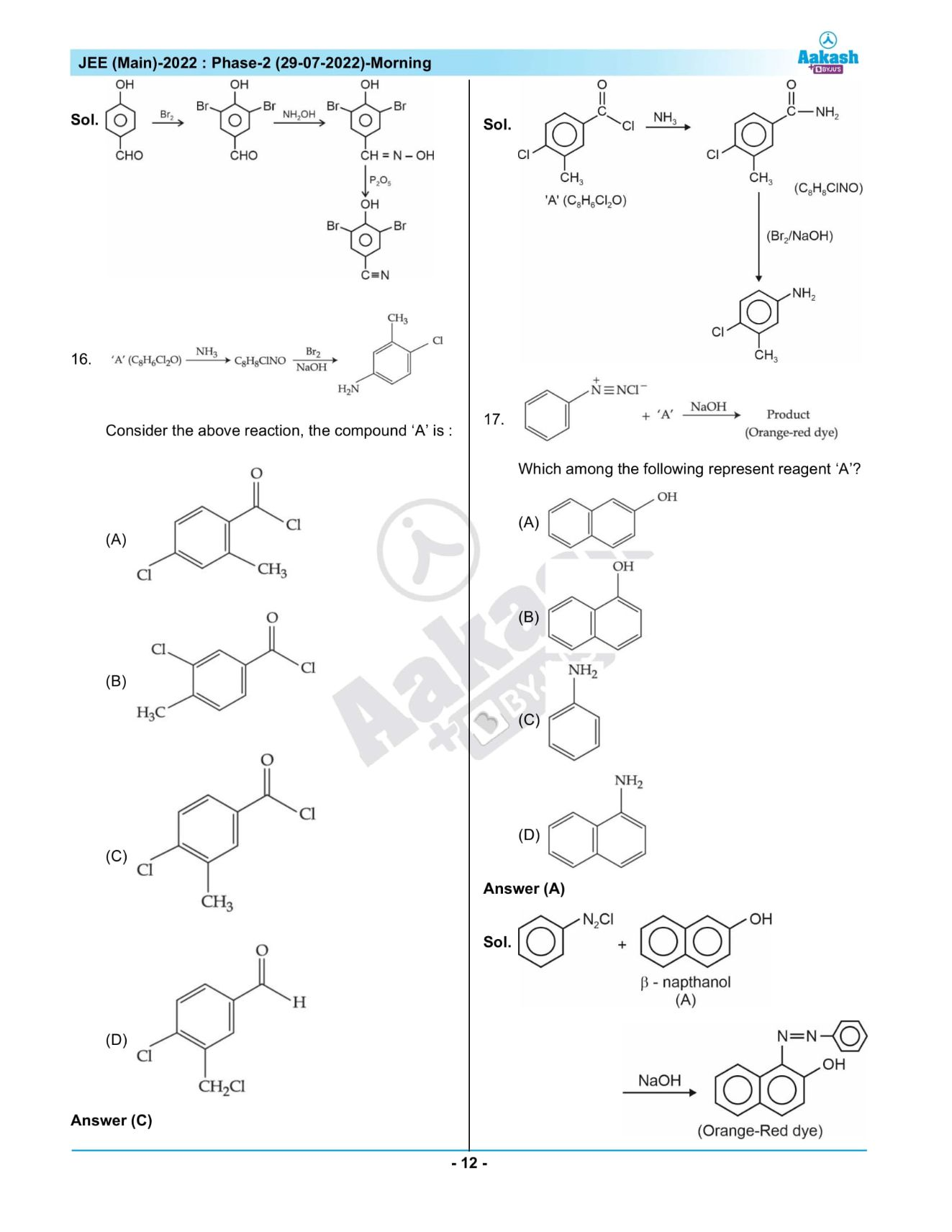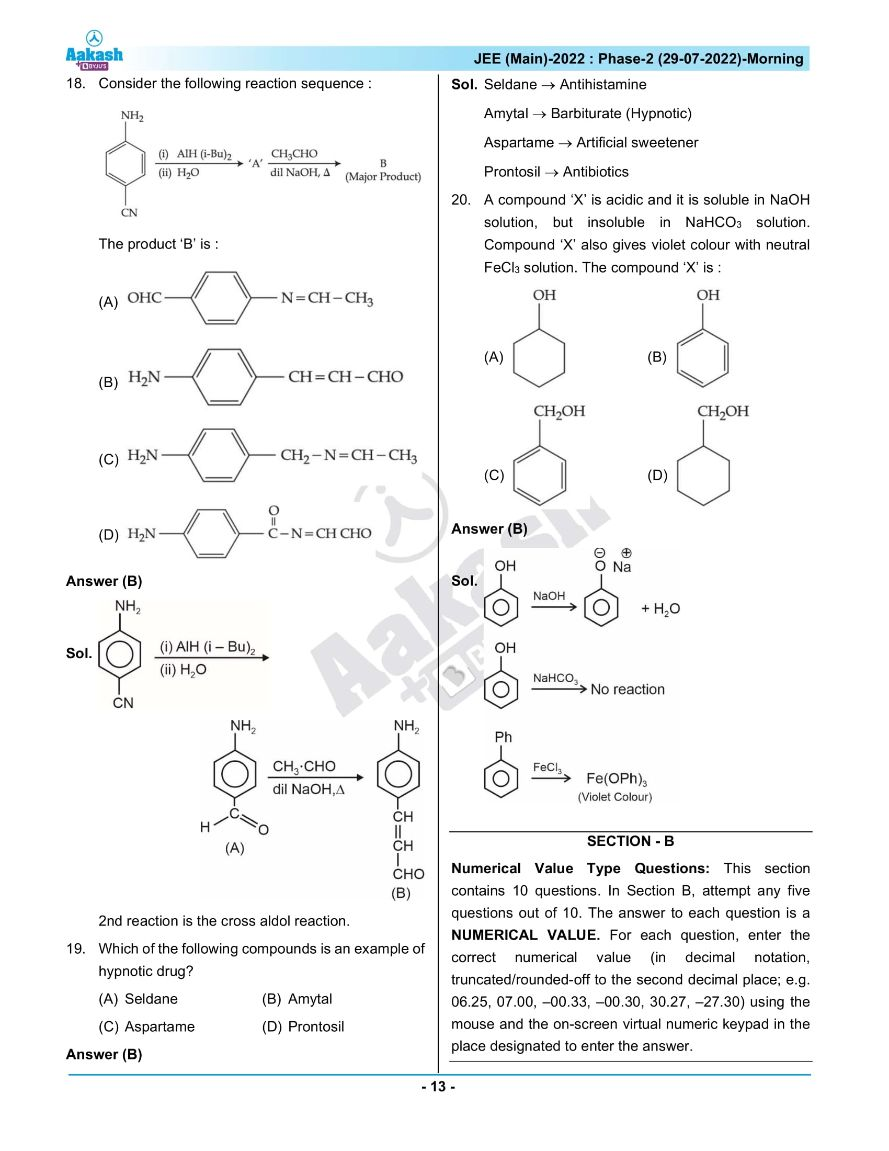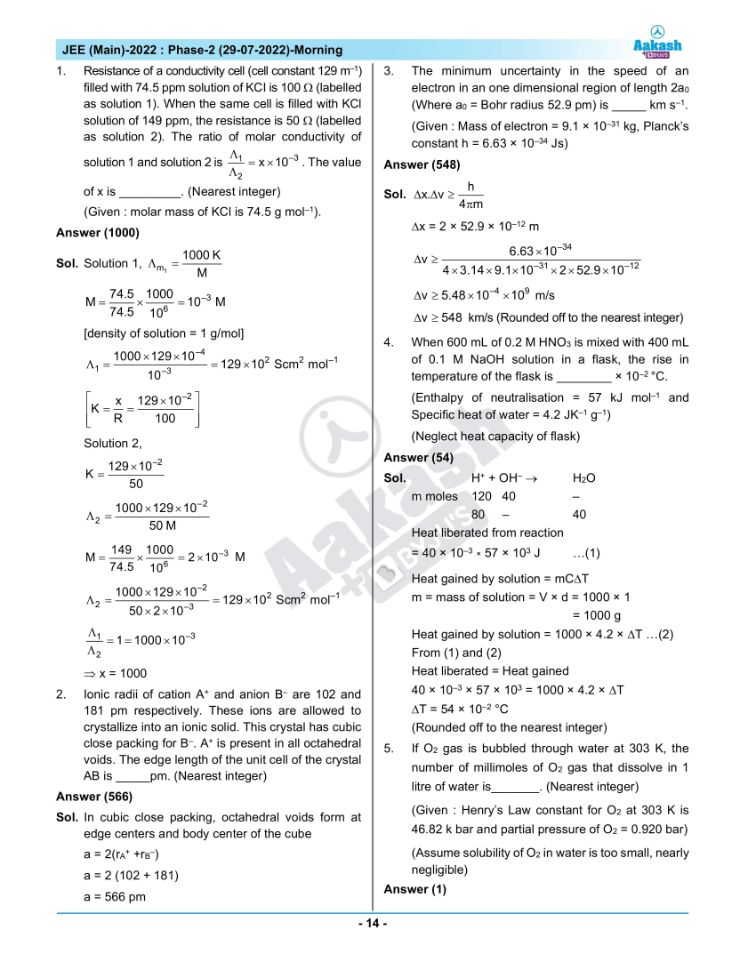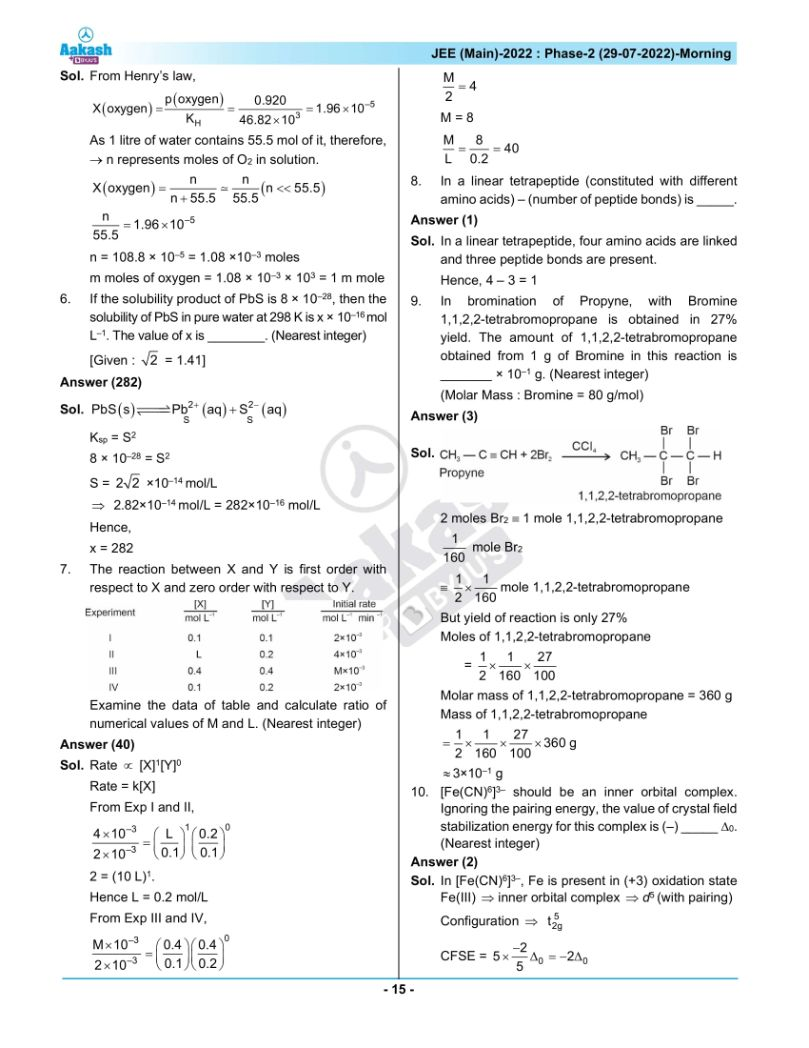## JEE Main 2022 July 29th Shift 1 Paper Analysis